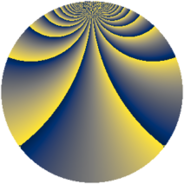# Properties

 Label 1960.2.sLevel $1960$ Weight $2$ Character orbit 1960.s Rep. character $\chi_{1960}(293,\cdot)$ Character field $\Q(\zeta_{4})$ Dimension $464$ Sturm bound $672$

# Learn more about

## Defining parameters

 Level: $$N$$ $$=$$ $$1960 = 2^{3} \cdot 5 \cdot 7^{2}$$ Weight: $$k$$ $$=$$ $$2$$ Character orbit: $$[\chi]$$ $$=$$ 1960.s (of order $$4$$ and degree $$2$$) Character conductor: $$\operatorname{cond}(\chi)$$ $$=$$ $$280$$ Character field: $$\Q(i)$$ Sturm bound: $$672$$

## Dimensions

The following table gives the dimensions of various subspaces of $$M_{2}(1960, [\chi])$$.

Total New Old
Modular forms 704 496 208
Cusp forms 640 464 176
Eisenstein series 64 32 32

## Trace form

 $$464q + 4q^{2} + 4q^{8} + O(q^{10})$$ $$464q + 4q^{2} + 4q^{8} - 16q^{15} + 24q^{16} + 32q^{18} - 16q^{22} + 8q^{23} + 8q^{25} + 64q^{30} + 44q^{32} - 48q^{36} - 40q^{46} + 68q^{50} - 40q^{57} + 92q^{58} - 12q^{60} + 8q^{65} + 32q^{71} - 24q^{72} + 72q^{78} - 288q^{81} + 48q^{86} - 24q^{88} + 12q^{92} + 40q^{95} + O(q^{100})$$

## Decomposition of $$S_{2}^{\mathrm{new}}(1960, [\chi])$$ into newform subspaces

The newforms in this space have not yet been added to the LMFDB.

## Decomposition of $$S_{2}^{\mathrm{old}}(1960, [\chi])$$ into lower level spaces

$$S_{2}^{\mathrm{old}}(1960, [\chi]) \cong$$ $$S_{2}^{\mathrm{new}}(280, [\chi])$$$$^{\oplus 2}$$